图像梯度_图像一阶梯度二阶梯度 - CSDN
• 学习图像处理的过程中，刚开始遇到图像梯度和一些算子的概念，这两者到底是什么关系，又有什么不同，一直困扰着我。后来在看到图像分割这一模块后才恍然大悟，其实图像的梯度可以用一阶导数和二阶偏导数来求解。但是...
                    图像的梯度（The Gradient of Image）

一、学习心得：

学习图像处理的过程中，刚开始遇到图像梯度和一些算子的概念，这两者到底是什么关系，又有什么不同，一直困扰着我。后来在看到图像分割这一模块后才恍然大悟，其实图像的梯度可以用一阶导数和二阶偏导数来求解。但是图像以矩阵的形式存储的，不能像数学理论中对直线或者曲线求导一样，对一幅图像的求导相当于对一个平面、曲面求导。对图像的操作，我们采用模板对原图像进行卷积运算，从而达到我们想要的效果。而获取一幅图像的梯度就转化为：模板（Roberts、Prewitt、Sobel、Lapacian算子）对原图像进行卷积，不过这里的模板并不是随便设计的，而是根据数学中求导理论推导出来的。下面就逐一分析各梯度算子的推导过程。

二、算子推导过程

1、知识引入：
在一维连续数集上有函数f(x),我们可以通过求导获得该函数在任一点的斜率，根据导数的定义有：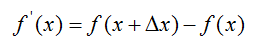在二维连续数集上有函数f(x,y),我们也可以通过求导获得该函数在x和y分量的偏导数，根据定义有：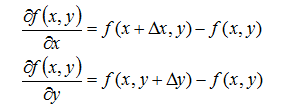2、梯度和Roberts算子：
对于图像来说，是一个二维的离散型数集，通过推广二维连续型求函数偏导的方法，来求得图像的偏导数，即在(x,y)处的最大变化率，也就是这里的梯度：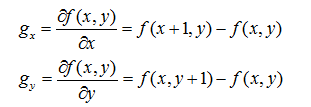梯度是一个矢量，则(x,y)处的梯度表示为：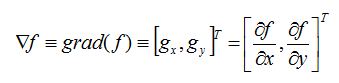其大小为：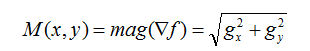因为平方和平方根需要大量的计算开销，所以使用绝对值来近似梯度幅值：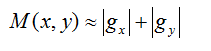方向与α(x,y)正交：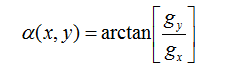其对应的模板为：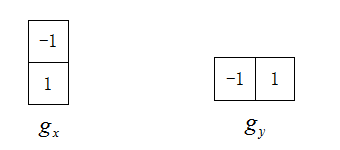上面是图像的垂直和水平梯度，但我们有时候也需要对角线方向的梯度，定义如下：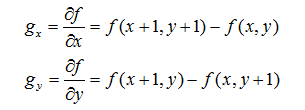对应模板为：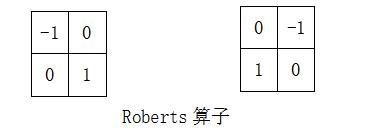上述模板就是Roberts交叉梯度算子。


1
2
2*2大小的模板在概念上很简单，但是他们对于用关于中心点对称的模板来计算边缘方向不是很有用，其最小模板大小为3*3。3*3模板考虑了中心点对段数据的性质，并携带有关于边缘方向的更多信息。

3、Prewitt和Sobel算子：
在3*3模板中：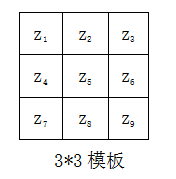我如下定义水平、垂直和两对角线方向的梯度：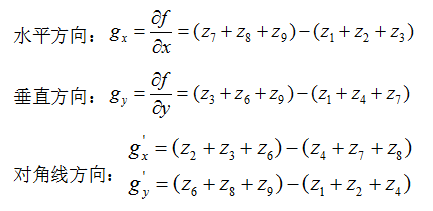该定义下的算子称之为Prewitt算子：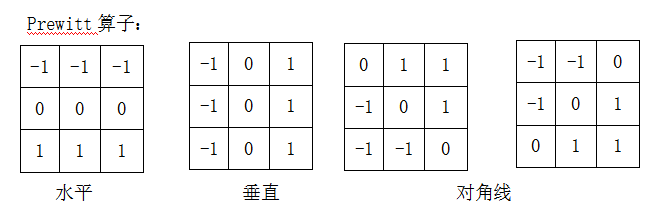Sobel算子是在Prewitt算子的基础上改进的，在中心系数上使用一个权值2，相比较Prewitt算子，Sobel模板能够较好的抑制（平滑）噪声。
计算公式为：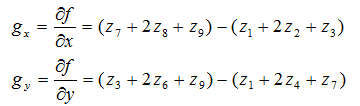Sobel算子：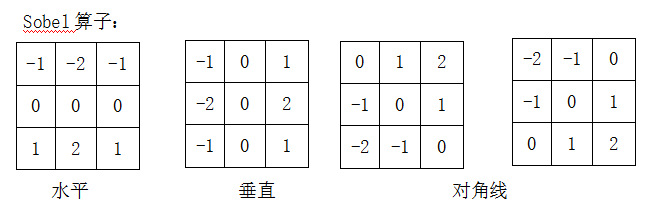上述所有算子都是通过求一阶导数来计算梯度的，用于线的检测，在图像处理中，通常用于边缘检测。在图像处理过程中，除了检测线，有时候也需要检测特殊点，这就需要用二阶导数进行检测。

4、Lapacian算子：
一阶导数：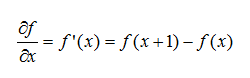二阶导数：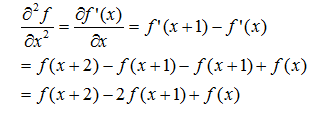我们感兴趣的是关于点x的二阶导数，故将上式中的变量减1后，得到：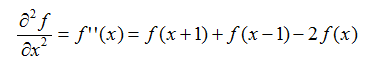在图像处理中通过拉普拉斯模板求二阶导数，其定义如下：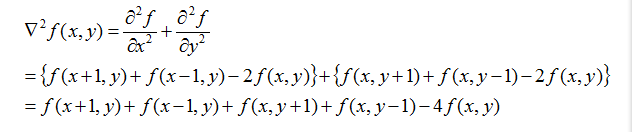对应模板为：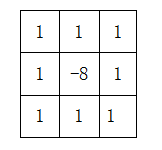模板中心位置的数字是-8而不是-4，是因为要使这些系数之和为0，当遇到恒定湖对区域时，模板响应应将0。

在用lapacian算子图像进行卷积运算时，当响应的绝对值超过指定阈值时，那么该点就是被检测出来的孤立点，具体输出如下：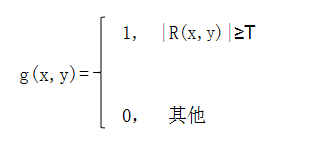索贝尔算子（Sobel operator）主要用作边缘检测，在技术上，它是一离散性差分算子，用来运算图像亮度函数的灰度之近似值。在图像的任何一点使用此算子，将会产生对应的灰度矢量或是其法矢量。

Sobel卷积因子为：该算子包含两组3x3的矩阵，分别为横向及纵向，将之与图像作平面卷积，即可分别得出横向及纵向的亮度差分近似值。如果以A代表原始图像，Gx及Gy分别代表经横向及纵向边缘检测的图像灰度值，其公式如下：具体计算如下：

Gx = (-1)*f(x-1, y-1) + 0*f(x,y-1) + 1*f(x+1,y-1)

+(-2)*f(x-1,y) + 0*f(x,y)+2*f(x+1,y)

+(-1)*f(x-1,y+1) + 0*f(x,y+1) + 1*f(x+1,y+1)

= [f(x+1,y-1)+2*f(x+1,y)+f(x+1,y+1)]-[f(x-1,y-1)+2*f(x-1,y)+f(x-1,y+1)]

Gy =1* f(x-1, y-1) + 2*f(x,y-1)+ 1*f(x+1,y-1)

+0*f(x-1,y) 0*f(x,y) + 0*f(x+1,y)

+(-1)*f(x-1,y+1) + (-2)*f(x,y+1) + (-1)*f(x+1, y+1)

= [f(x-1,y-1) + 2f(x,y-1) + f(x+1,y-1)]-[f(x-1, y+1) + 2*f(x,y+1)+f(x+1,y+1)]

其中f(a,b), 表示图像(a,b)点的灰度值；

图像的每一个像素的横向及纵向灰度值通过以下公式结合，来计算该点灰度的大小：通常，为了提高效率 使用不开平方的近似值：如果梯度G大于某一阀值 则认为该点(x,y)为边缘点。

然后可用以下公式计算梯度方向：Sobel算子根据像素点上下、左右邻点灰度加权差，在边缘处达到极值这一现象检测边缘。对噪声具有平滑作用，提供较为精确的边缘方向信息，边缘定位精度不够高。当对精度要求不是很高时，是一种较为常用的边缘检测方法。

普利维特算子(Prewitt operate)：

除sobel边缘检测外 还有Prewitt算子， 它的卷积因子如下：其他计算 和sobel差不多；

Prewitt算子利用像素点上下、左右邻点灰度差，在边缘处达到极值检测边缘。对噪声具有平滑作用，定位精度不够高。

罗伯茨交叉边缘检测（Roberts Cross operator）

卷积因子如下：灰度公式为：近似公式为：具体计算如下：

G(x,y)=abs(f(x,y)-f(x+1,y+1))+abs(f(x,y+1)-f(x+1,y))

灰度方向 计算公式为：Roberts算子采用对角线方向相邻两像素之差近似梯度幅值检测边缘。检测水平和垂直边缘的效果好于斜向边缘，定位精度高，对噪声敏感。

【转载】：https://blog.csdn.net/liuxiangxxl/article/details/78226617?locationNum=4&fps=1

https://blog.csdn.net/image_seg/article/details/78790968


展开全文• 1.图像梯度的定义图像函数f(x,y)在点(x,y)的梯度是一个具有大小和方向的矢量，设为Gx 和 Gy 分别表示x方向和y方向的梯度，这个梯度的矢量可以表示为：图像梯度：G(x,y)=dx i +dy j;dx(i,j)=Img(i+1,j)-I...
图像在计算机中以数字图像的形式存储，即以数值矩阵的形式存在，形成了离散的数值信号，在此基础上，对于图像处理中的数值的多样性计算分析也影响着初步图像分析。图像梯度的定义：图像函数f(x,y)在点(x,y)的梯度是一个具有大小和方向的矢量，设为Gx 和 Gy 分别表示x方向和y方向的梯度，这个梯度的矢量可以表示为：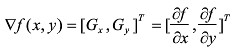图像梯度：G(x,y)=dx i +dy j;dx(i,j)=Img(i+1,j)-Img(i,j);dy(i,j)=Img(i,j+1)-I(i,j);Img(i,j)是图像数值矩阵的像素值，（i，j）为像素相应坐标。Gradient(Img)函数求的是数值上的梯度，假设Img为图片数值矩阵.Matlab中计算方法: [Rx,Ry]=gradient(Img)；(1)水平方向梯度Rx，Rx的第一列元素为原矩阵第二列与第一列元素之差，Rx的第二列元素为原矩阵第三列与第一列元素之差除以2，以此类推：Fx(i,j)=(F(i,j+1)-F(i,j-1))/2。最后一列则为最后两列之差。(2)垂直方向梯度Ry，同理，可以得到Fy。
展开全文• 前面我们提到，当用均值滤波器降低图像噪声的时候，会带来图像模糊的副作用。我们当然希望看到的是清晰图像。那么，清晰图像和模糊图像之间的差别在哪里呢？从逻辑上考虑，图像模糊是因为图像中物体的轮廓不明显，...
前面我们提到，当用均值滤波器降低图像噪声的时候，会带来图像模糊的副作用。我们当然希望看到的是清晰图像。那么，清晰图像和模糊图像之间的差别在哪里呢？从逻辑上考虑，图像模糊是因为图像中物体的轮廓不明显，轮廓边缘灰度变化不强烈，层次感不强造成的，那么反过来考虑，轮廓边缘灰度变化明显些，层次感强些是不是图像就更清晰些呢。

那么，这种灰度变化明显不明显怎样去定义呢。我们学过微积分，知道微分就是求函数的变化率，即导数（梯度），那么对于图像来说，可不可以用微分来表示图像灰度的变化率呢，当然是可以的，前面我们提到过，图像就是函数嘛。

在微积分中，一维函数的一阶微分的基本定义是这样的：

dfdx=limϵ→0f(x+ϵ)−f(x)ϵ
\frac{df}{dx}=\lim_{\epsilon\rightarrow 0 }{\frac{f(x+\epsilon )-f(x)}{\epsilon }}

而图像是一个二维函数f(x,y)，其微分当然就是偏微分。因此有：

∂f(x,y)∂x=limϵ→0f(x+ϵ,y)−f(x,y)ϵ\frac{\partial f(x,y)}{\partial x}=\lim_{\epsilon\rightarrow 0}{\frac{f(x+\epsilon,y )-f(x,y)}{\epsilon }}

∂f(x,y)∂y=limϵ→0f(x,y+ϵ)−f(x,y)ϵ
\frac{\partial f(x,y)}{\partial y}=\lim_{\epsilon\rightarrow 0}{\frac{f(x,y+\epsilon)-f(x,y)}{\epsilon }}

因为图像是一个离散的二维函数，ϵ$\epsilon$不能无限小，我们的图像是按照像素来离散的，最小的ϵ$\epsilon$就是1像素。因此，上面的图像微分又变成了如下的形式（ϵ=1$\epsilon=1$）：

∂f(x,y)∂x=f(x+1,y)−f(x,y)=gx
\frac{\partial f(x,y)}{\partial x}=f(x+1,y)-f(x,y)=gx

∂f(x,y)∂y=f(x,y+1)−f(x,y)=gy
\frac{\partial f(x,y)}{\partial y}=f(x,y+1)-f(x,y)=gy

这分别是图像在(x, y)点处x方向和y方向上的梯度，从上面的表达式可以看出来，图像的梯度相当于2个相邻像素之间的差值。

那么，这个梯度（或者说灰度值的变化率）如何增强图像的清晰度呢？

我们先考虑下x方向，选取某个像素，假设其像素值是100，沿x方向的相邻像素分别是90,90,90，则根据上面的计算其x方向梯度分别是10,0,0。这里只取变化率的绝对值，表明变化的大小即可。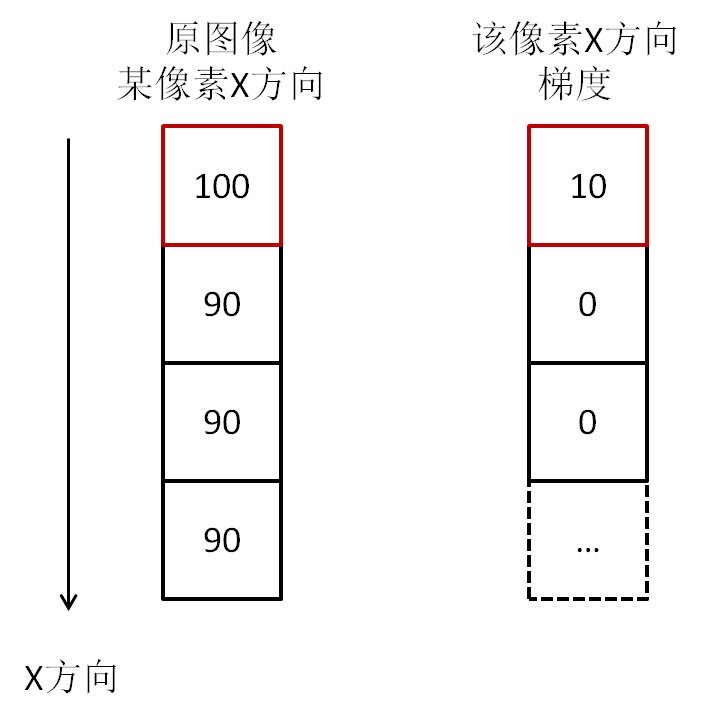我们看到，100和90之间亮度相差10，并不是很明显，与一大群90的连续灰度值在一起，轮廓必然是模糊的。我们注意到，如果相邻像素灰度值有变化，那么梯度就有值，如果相邻像素灰度值没有变化，那么梯度就为0。如果我们把梯度值与对应的像素相加，那么灰度值没有变化的，像素值不变，而有梯度值的，灰度值变大了。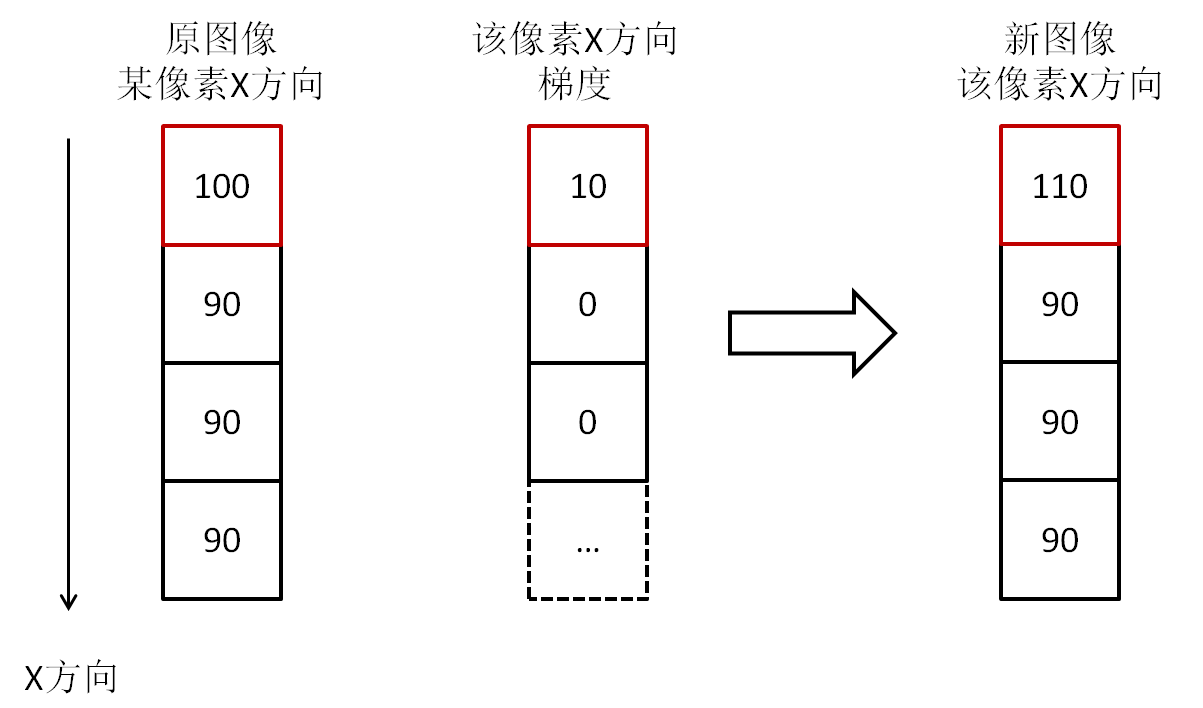我们看到，相加后的新图像，原图像像素点100与90亮度只相差10，现在是110与90，亮度相差20了，对比度显然增强了，尤其是图像中物体的轮廓和边缘，与背景大大加强了区别，这就是用梯度来增强图像的原理。

上面只是说了x方向，y方向是一样的。那么能否将x方向和y方向的梯度结合起来呢？当然是可以的。x方向和y方向上的梯度可以用如下式子表示在一起：
M(x,y)=(gx)2+(gy)2−−−−−−−−−−−√
M(x,y)=\sqrt{(gx)^{2}+(gy)^{2}}

这里又是平方，又是开方的，计算量比较大，于是一般用绝对值来近似平方和平方根的操作，来降低计算量：

M(x,y)=|gx|+|gy|
M(x,y)=\left |gx  \right |+\left | gy \right |

我们来计算一下月球图像的x方向和y方向结合的梯度图像，以及最后的增强图像。(原图像来自冈萨雷斯的《数字图像处理》一书。)

import cv2
import numpy as np

row, column = moon.shape
moon_f = np.copy(moon)
moon_f = moon_f.astype("float")

for x in range(row - 1):
for y in range(column - 1):
gx = abs(moon_f[x + 1, y] - moon_f[x, y])
gy = abs(moon_f[x, y + 1] - moon_f[x, y])
gradient[x, y] = gx + gy

sharp = np.where(sharp < 0, 0, np.where(sharp > 255, 255, sharp))

sharp = sharp.astype("uint8")
cv2.imshow("moon", moon)
cv2.imshow("sharp", sharp)
cv2.waitKey()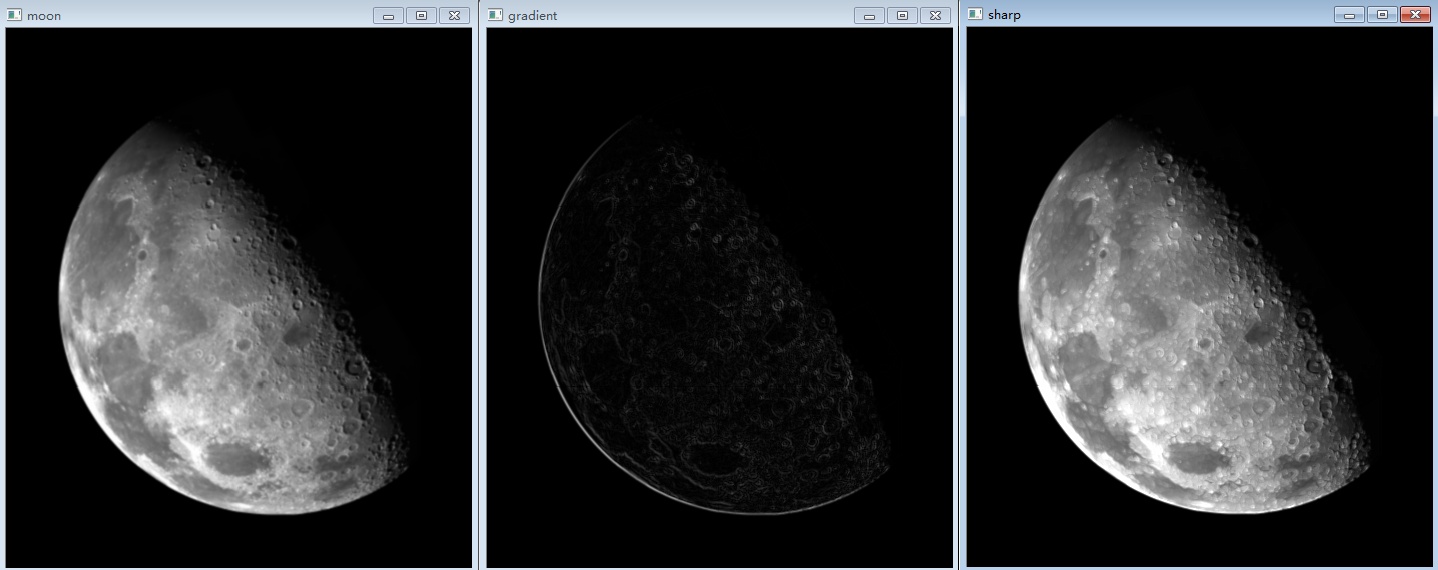展开全文图像处理 opencv 图像梯度
• 一、图像梯度的定义 图像函数f(x,y)在点(x,y)的梯度是一个具有大小和方向的矢量，设为Gx 和 Gy 分别表示x方向和y方向的梯度，这个梯度的矢量可以表示为：   这个矢量的幅度为   方向角为：   对于...
一、图像梯度的定义

图像函数f(x,y)在点(x,y)的梯度是一个具有大小和方向的矢量，设为Gx 和 Gy 分别表示x方向和y方向的梯度，这个梯度的矢量可以表示为：这个矢量的幅度为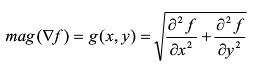方向角为：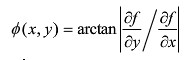对于数字图像而言，相当于对二维离散函数求梯度，如下：

G(x,y) = dx(i,j) + dy(i,j);

dx(i,j)  = I(i+1,j) - I(i,j);

dy(i,j)  = I(i,j+1) - I(i,j);

数字图像中，更多的使用差分来近似导数，最简单的梯度近似表达式如下：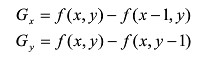梯度的方向是函数f(x,y)变化最快的方向，当图像中存在边缘时，一定有较大的梯度值，相反，当图像中有比较平滑的部分时，灰度值变化较小，则相应的梯度也较小，图像处理中把梯度的模简称为梯度，由图像梯度构成的图像成为梯度图像(we can get the gradient image through computing the gradient of each pixel in the image)

经典的图像梯度算法是考虑图像的每个像素的某个邻域内的灰度变化，利用边缘临近的一阶或二阶导数变化规律，对原始图像中像素某个邻域设置梯度算子，通常我们用小区域模板进行卷积来计算，有Sobel算子、Robinson算子、Laplace算子等。

二、图像一阶微分应用

首先看一下数学中一维的微分公式Δf = f(x+1) – f(x), 对于一幅二维的数字图像f(x,y)而言，需要完

成XY两个方向上的微分，所以有如下的公式：分别对X,Y两个方向上求出它们的偏微分，最终得到梯度Delta F.

对于离散的图像来说,一阶微分的数学表达相当于两个相邻像素的差值，根据选择的梯度算

子不同，效果可能有所不同，但是基本原理不会变化。最常见的算子为Roberts算子，其它

常见还有Sobel，Prewitt等算子。以Roberts算子为例的X,Y的梯度计算演示如下图：图像微分(梯度计算)是图像边缘提取的重要的中间步骤，根据X,Y方向的梯度向量值，可以

得到如下两个重要参数振幅magnitude, 角度theta，计算公式如下：magnitude表示边缘强度信息

theta预言边缘的方向走势。

假如对一幅数字图像，求出magnitude之后与原来每个像素点对应值相加，则图像边缘将被

大大加强，轮廓更加明显，是一个很典型的sharp filter的效果。

转载自：https://blog.csdn.net/image_seg/article/details/78790968
展开全文图像处理
• 文章目录一、梯度二、图像梯度 一、梯度 数学梯度：是一个向量，由一组正交的方向导数组成，表示函数在该点处的方向导数沿着该方向取得最大值，即函数在该点处沿着该方向变化最快，变化最大。 它的基本特性指出了...图像梯度
• 前面我们提到，当用均值滤波器降低图像噪声的时候，会带来图像模糊的副作用。我们当然希望看到的是清晰图像。那么，清晰图像和模糊图像之间的差别在哪里呢？从逻辑上考虑，图像模糊是因为图像中物体的轮廓不明显，...
• 连续函数在某一点的导数可以由 得到，图像是离散函数，在某点的梯度可以用向前差商、向后差商或者中心差商获得。这里采用中心差商 可以获取图像某点的导数值。计算过程如下图所示 写成一维卷积的形式等于与如下...
• 由于图像在计算机中以数字图像的形式进行 存储， 即图像是离散的数字信号， 对数字图像梯度使用差分来代替连续信号中 的微分。 由于图像在计算机中以数字图像的形式进行 存储， 即图像是离散的数字信号， ...
• 图像梯度域融合 图像梯度域重建 图像泊松编辑 混合梯度融合 彩色图像灰度化的问题 图像梯度域重建-原理 该问题中心思想是利用梯度实现灰度图像的重建。为后面的问题作铺垫。 该问题用到了梯度与最小二乘的思想。 ...
• 图像梯度可以把图像看成二维离散函数，图像梯度其实就是这个二维离散函数的求导： 图像梯度: G(x,y) = dx(i,j) + dy(i,j); dx(i,j) = I(i+1,j) - I(i,j); dy(i,j) = I(i,j+1) - I(i,j); 其中，I是图像...
• 利用图像梯度损失约束网络能够更好的在图像生成过程中产生更加清晰的纹理细节，但是如何利用pytorch计算图像梯度并作为梯度损失可能在网络优化过程中造成梯度爆炸。本文将阐述如何利用pytorch计算图像梯度并作为图像...pytorch 深度学习 神经网络 自编码器
• 图像处理之图像梯度效果 基本思想： 利用X方向与Y方向分别实现一阶微分，求取振幅，实现图像梯度效果。关于如何计算图像 一阶微分参见这里：http://blog.csdn.net/jia20003/article/details/7562092 使用的两种...图像处理 null filter date class
• **图像梯度的概念：** 详情请看：[图像梯度的基本原理](https://blog.csdn.net/saltriver/article/details/78987096) 重点在于：图像的梯度和函数的梯度概念差不多，图像可以看做是离散的函数，每个离散点即为像素...Matlab 实验室 图像处理
• 图像梯度算子简介 　相信只要是懂些图像处理的知识，都知道图像梯度的含义。不知道是否考虑过为什么图像梯度如此广泛认知与使用？为什么不使用图像纹理、图像色彩、图像相位等等，在这里我并不是说上述除了图像梯度...sobel laplacian 图像梯度
• 前面我们介绍过图像的梯度，其定义是根据微积分的定义在二维离散函数中推导出来的。但是，我们需要理解，梯度只是一个工具，方法，核心目的是得到像素点...那么，根据图像梯度的定义： gx = z8 - z5 gy = z6 - z5...
• 文章目录图像梯度的定义（离散）图像梯度理解 图像梯度的定义（离散） 对于一个二元函数F(x,y)F(x,y)F(x,y)来说，其偏导数的定义为： δF(x,y)δx=lim⁡ϵ→0F(x+ϵ,y)−F(x,y)ϵ\frac {\delta F(x,y)}{\delta x}=\...
• 图像梯度求解的理论知识 连续函数在某一点的导数可以由 得到。 图像是离散函数，在某点的梯度可以用向前差商、向后差商或者中心差商获得。这里采用中心差商 可以获取图像某点的导数值。计算过程如下图所示 ...
• 简介：图像梯度可以把图像看成二维离散函数，图像梯度其实就是这个二维离散函数的求导。 Sobel算子是普通一阶差分，是基于寻找梯度强度。拉普拉斯算子（二阶差分）是基于过零点检测。通过计算梯度，设置阀值，得到...
• 特征描述符与图像梯度直方图 特征描述符就是通过提取图像的有用信息，并且丢弃无关信息来简化图像的表示； 梯度直方图（Histogram of Gradients, HOG） 1. 将图像按照设定比例分成若干cell，比如1600*3200的图像...
• OpenCV图像梯度之Sobel算子0.综述2.Sobel算子原理分析3.Sobel算子OpenCV API介绍4.Sobel算子代码实践 0.综述 Sobel算子是提取图像梯度信息的经典算子之一，图像的梯度信息是图像的最原始特征信息，对梯度信息进行...
...